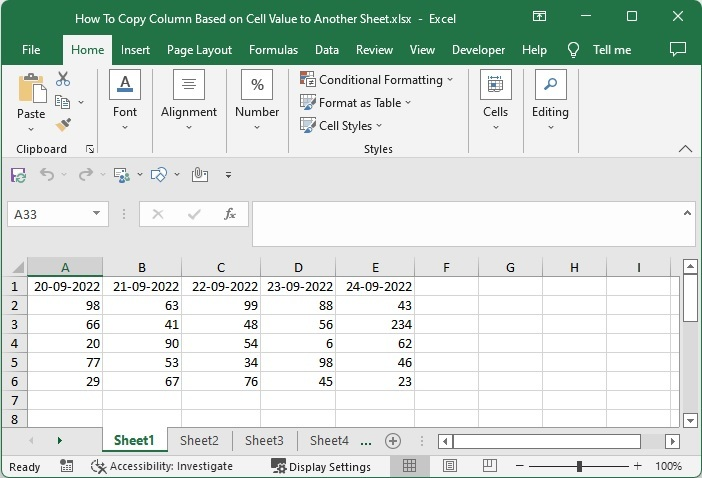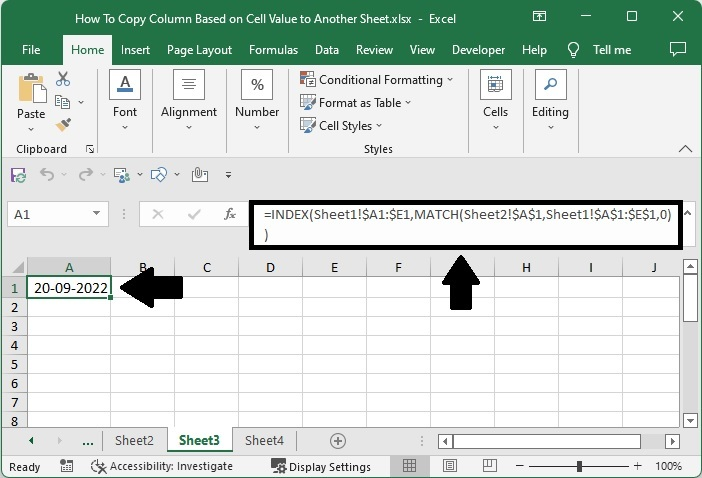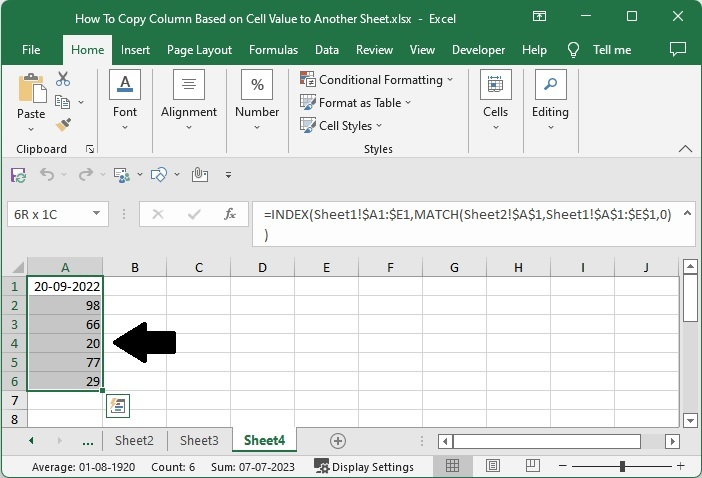# How to Copy a Column Based on Cell Value to Another Excel Sheet?

Have you ever tried to copy a column based on a single cell value to another sheet in Excel, i.e., copied a whole column using a cell value as a reference? We can use the process mentioned in this tutorial to copy the whole column using a single cell of the column.

Read this tutorial to learn how to copy a column based on cell value to another sheet in Excel. We can complete this using the INDEX function and the cell address. If we try to complete this task manually, we need to search for every single value based on a cell value.

## Copy a Column Based on Cell Value in Excel

Here we will use the formula to get any one of the results, then use the autofill handler to complete our task. Let's go over a simple procedure for copying a column based on cell value to another sheet in Excel using the INDEX function. We will have data on the first sheet, cell values on the second sheet, and print on the third sheet.

### Step 1

Consider an excel sheet where the data is represented as a collection of lists, as shown in the image below. We have to make sure that the header of the column is uniqueNow click on an empty cell on the other sheet and enter the formula as

=INDEX(Sheet1!$A1:$E1,MATCH(Sheet2!$A$1,Sheet1!$A$1:$E$1,0)) and click Enter to get our header value, similar to the below image. In the formula, A1:E1 is the range of cell values, and A1 is the address of our reference to copy.### Step 2

Now drag down from the header that we got in the above step using the auto-fill handle till zeros are printed as a result, then delete the excess zeros, and the final result will be similar to the below image.## Conclusion

In this tutorial, we used a simple example to demonstrate how you can copy a column based on a cell value to another sheet in Excel.